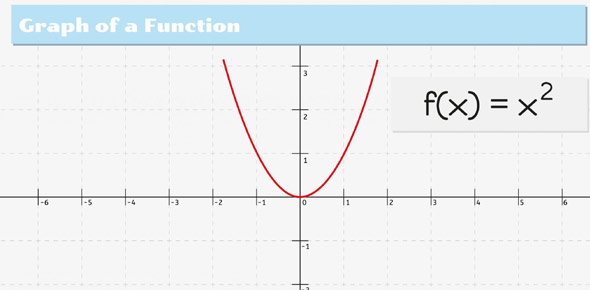# Mt 3 12-05-04 Proportional And Non-proportional Functions

22 Questions | Total Attempts: 60SettingsCreate your own Quiz• 1.
1. Is this proportional?
• A.

Yes

• B.

No

• 2.
1. If it is proportional or if you want a challenge, what is the rate of change (dollars/point)?  Round to the nearest ten-thousandth (4 decimal digits).  Do not include the \$ symbol.  If it is not proportional, enter 0.
• 3.
2.  Is it proportional?
• A.

Yes

• B.

No

• 4.
2. If it is proportional or if you want a challenge, whatis the rate of change (dollars/point)? Round to the nearest ten-thousandth (4 decimal digits).  Do not include the \$ symbol.  If it is not proportional, enter 0.
• 5.
3.  Is it proportional?
• A.

Yes

• B.

No

• 6.
3. If it is proportional or if you want a challenge, whatis the rate of change (dollars/ticket)?  There shouldn't be any decimal digits.  Do not include the \$ symbol.  If it is not proportional, enter 0.
• 7.
3. If it is proportional or if you want a challenge, write an equation in the form of c = ..., using n for the independent variable and with a space on each sided of the equals sign.  There shouldn't be any parentheses.  If it is not proportional, enter 0.
• 8.
4. Is this proportional?
• A.

Yes

• B.

No

• 9.
4. If it is proportional or if you want a challenge, what is the rate of change (dollars/mile)?  Do not include the \$ symbol.  If it is not proportional, enter 0.
• 10.
4.  If it is proportional or if you want a challenge, write the equation in the form of c = ..., using m for the independent variable and with a space on each sided of the equals sign, no parentheses.  Put the term with m before the constant term.  If it is not proportional, you may enter 0.
• 11.
5.  Is it proportional?
• A.

Yes

• B.

No

• 12.
5.  If it is proportional or if you want a challenge, what is the rate of change (miles/hour)?  If it is not proportional, you may enter 0.
• 13.
5. If it is proportional or if you want a challenge, write the equation in the form h = ..., using t as the independent variable.  If it is not proportional, you may enter 0.
• 14.
6. Is this proportional?
• A.

Yes

• B.

No

• 15.
6.  If it is proportional or if you want a challenge, what is the rate of change (miles/hour)?  If it is not proportional, you may enter 0.
• 16.
6.  If it is proportional or if you want a challenge, write equation in the form r = ..., using t as the other variable.  If it is not proportional, you may enter 0.
• 17.
7. Is this proportional?
• A.

Yes

• B.

No

• 18.
7.  If it is proportional or if you want a challenge, what is the rate of change (dollars/minutes)?  If it is not proportional, you may enter 0.
• 19.
7.  If it is proportional or if you want a challenge, write the equation in the form c = ..., using m as the other variable.  If it is not proportional, you may enter 0.
• 20.
8.  Is it proportional?
• A.

Yes

• B.

No

• 21.
8.  If it is proportional or if you want a challenge, what is the rate of change (dollars/minutes, no dollar sign)?  If it is not proportional, you may enter 0.
• 22.
8. If it is proportional or if you want a challenge, write the equation in the form d = ..., using m as the other variable.  If it is not proportional, you may enter 0.
Related TopicsBack to top
×

Wait!
Here's an interesting quiz for you.